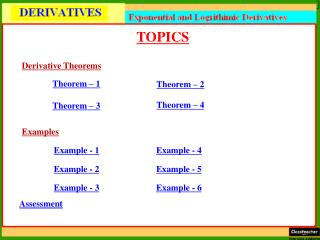DownloadDownload PresentationTOPICS

# TOPICS

Download Presentation## TOPICS

- - - - - - - - - - - - - - - - - - - - - - - - - - - E N D - - - - - - - - - - - - - - - - - - - - - - - - - - -
##### Presentation Transcript

1. TOPICS Derivative Theorems Theorem – 1 Theorem – 2 Theorem – 4 Theorem – 3 Examples Example - 1 Example - 4 Example - 2 Example - 5 Example - 3 Example - 6 Assessment

2. Theorem – 1: Proof:

3. Theorem – 2: Proof:

4. Theorem – 3: Proof:

5. Theorem – 4: Proof:

6. Example – 1: Solution:

7. Example – 2: Solution: Cont…

8. Example – 3:Differentiate log sin x by first principles. Solution: Cont…

9. Example – 4: Solution:

10. Example – 5:Differentiate the following function with respect to x: Solution:

11. Example – 6:Differentiate the following function with respect to x: Solution:

12. ASSESSMENT Differentiate the following by first principles: I. e–x II. x2ex III. x sin x log x Differentiate the following with respect to x: I. x2ex log x • II. xnlogax ANSWERS

13. ANSWERS Differentiate the following by first principles: • I. – e–x • II. (x2+ 2x )ex III. Differentiate the following with respect to x: • I. xex(1 + x log x + 2 log x) • II. xn –1(nlogax + 1/loga)